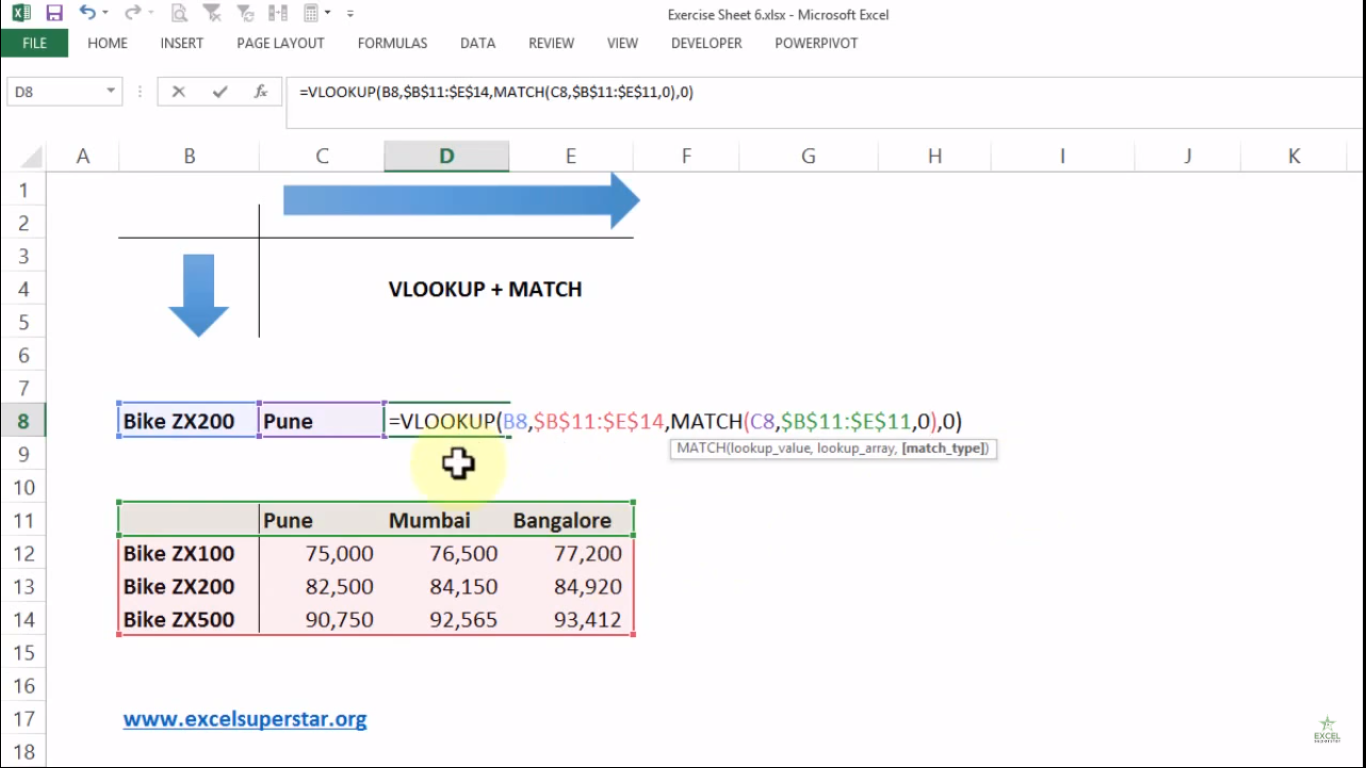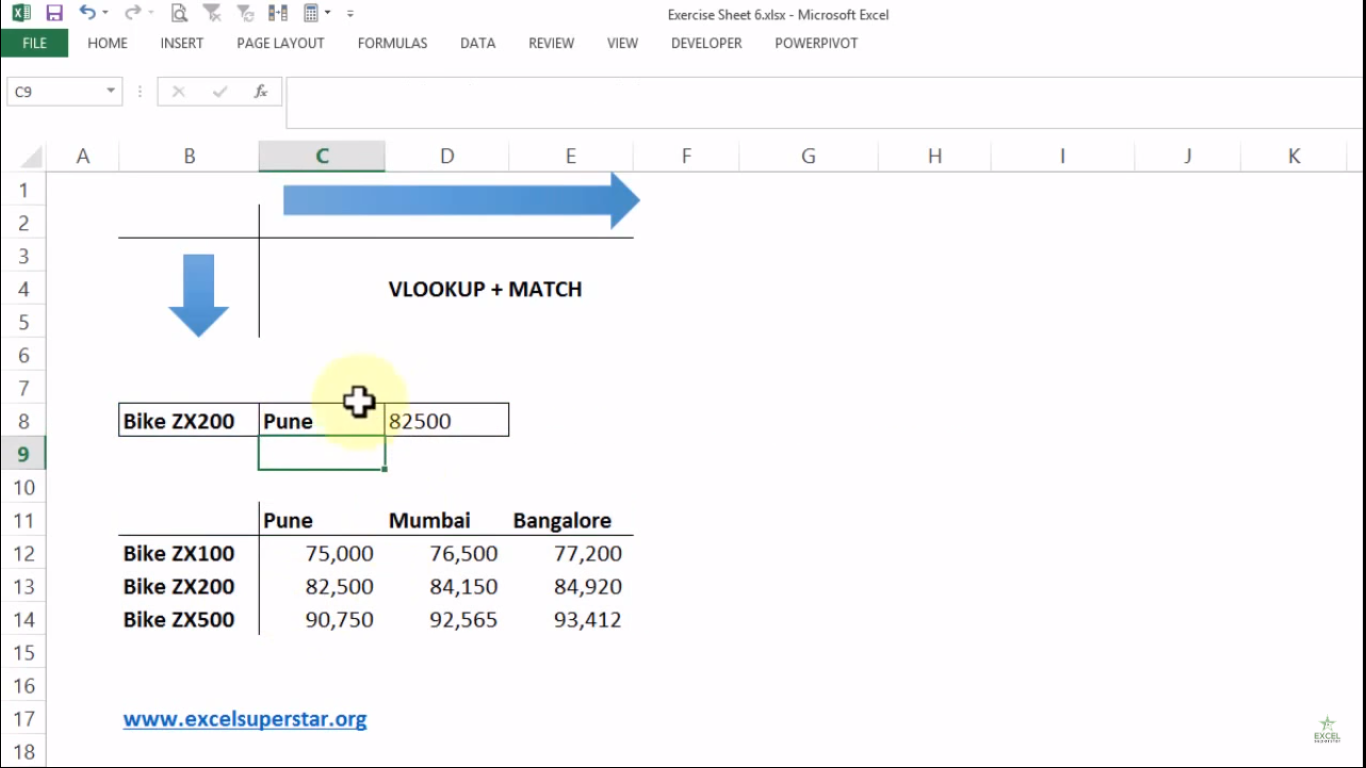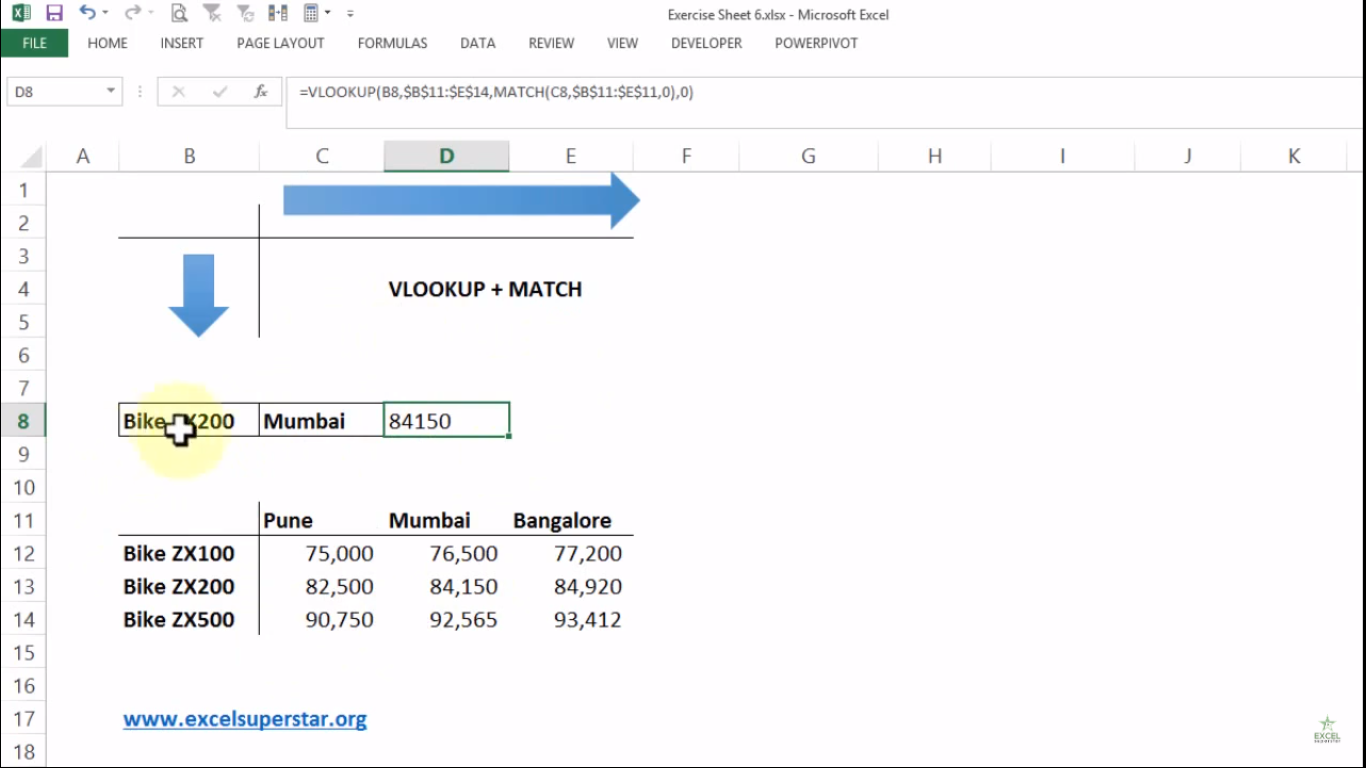# Advanced Excel VLOOKUP Formula with MatchIn this tutorial we will learn about Using VLOOKUP with MATCH for 2d lookup in Advanced Excel with the help of an example given below:

ExampleAssume that you a have price card for different bike models, and the prices of Bike model is different in various cities like Mumbai, Pune, Bangalore

So here there are 2 variables.

1. Bike Models from top to bottom

2. Name of the cities

Now you want to find out the price of Bike ZX 200 model in Mumbai city from the given table

So to find out the price, we will be applying the 2d VLOOKUP Formula by following the steps given below:

1. Press =VLOOKUP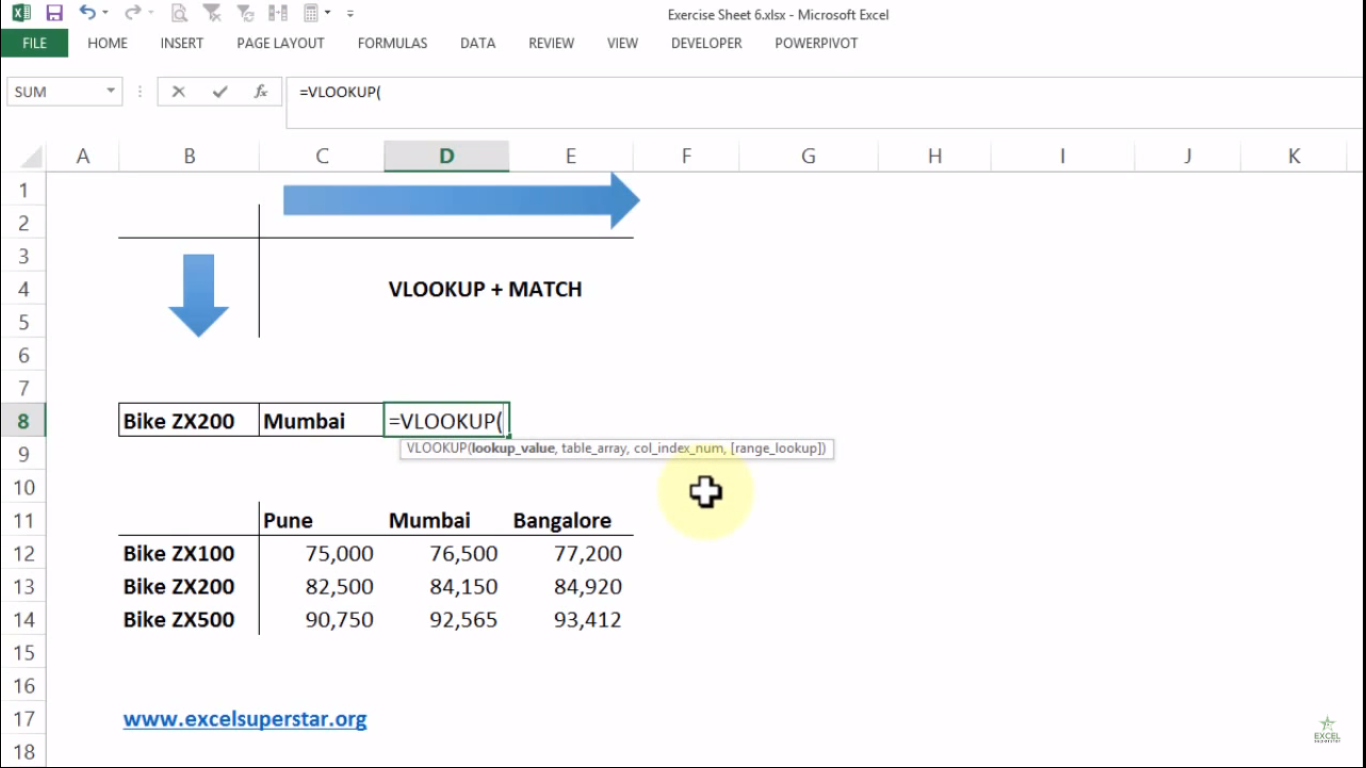Now there are 4 parameters (lookup_value, table_array, col_index_num, [range_lookup])

The first parameter lookup_value means the value to look for in the first column of a table.

2. Choose Bike ZX 200 cell which is given vertically in the table =VLOOKUP(B8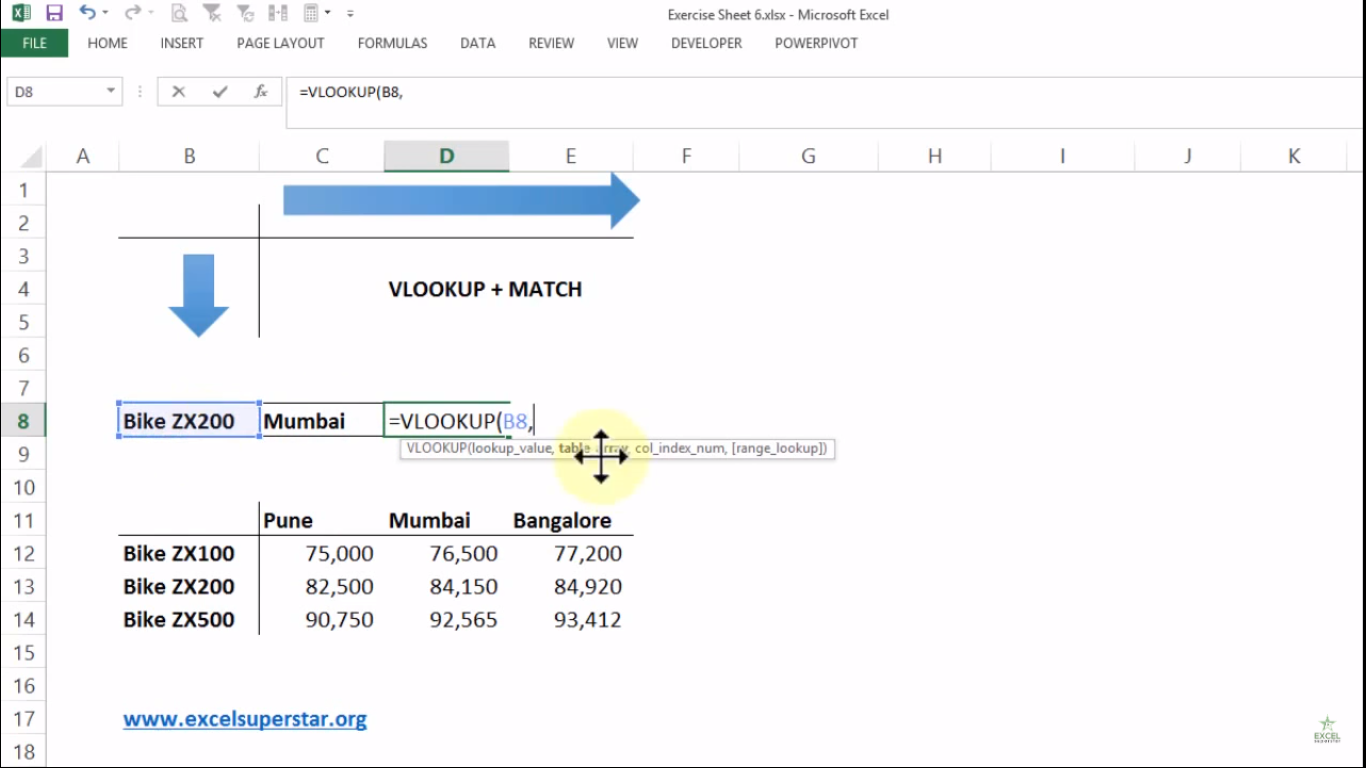The second parameter table_array means the table from which to retrieve a value.

3. Select the entire table and press F4 =VLOOKUP(B8,\$B\$11:\$E\$14,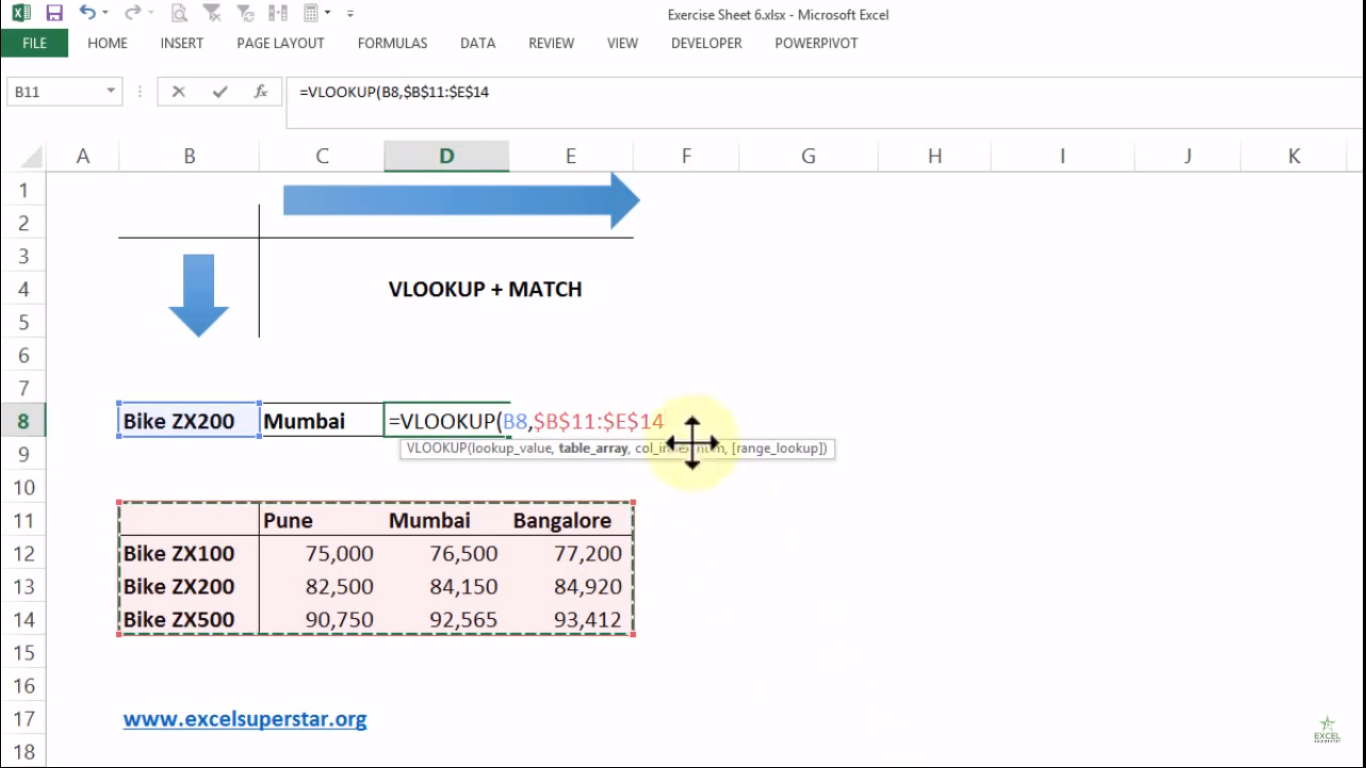The third parameter col_index_num means the column in the table from which to retrieve a value.

4. Write 3 =VLOOKUP(B8,\$B\$11:\$E\$14,3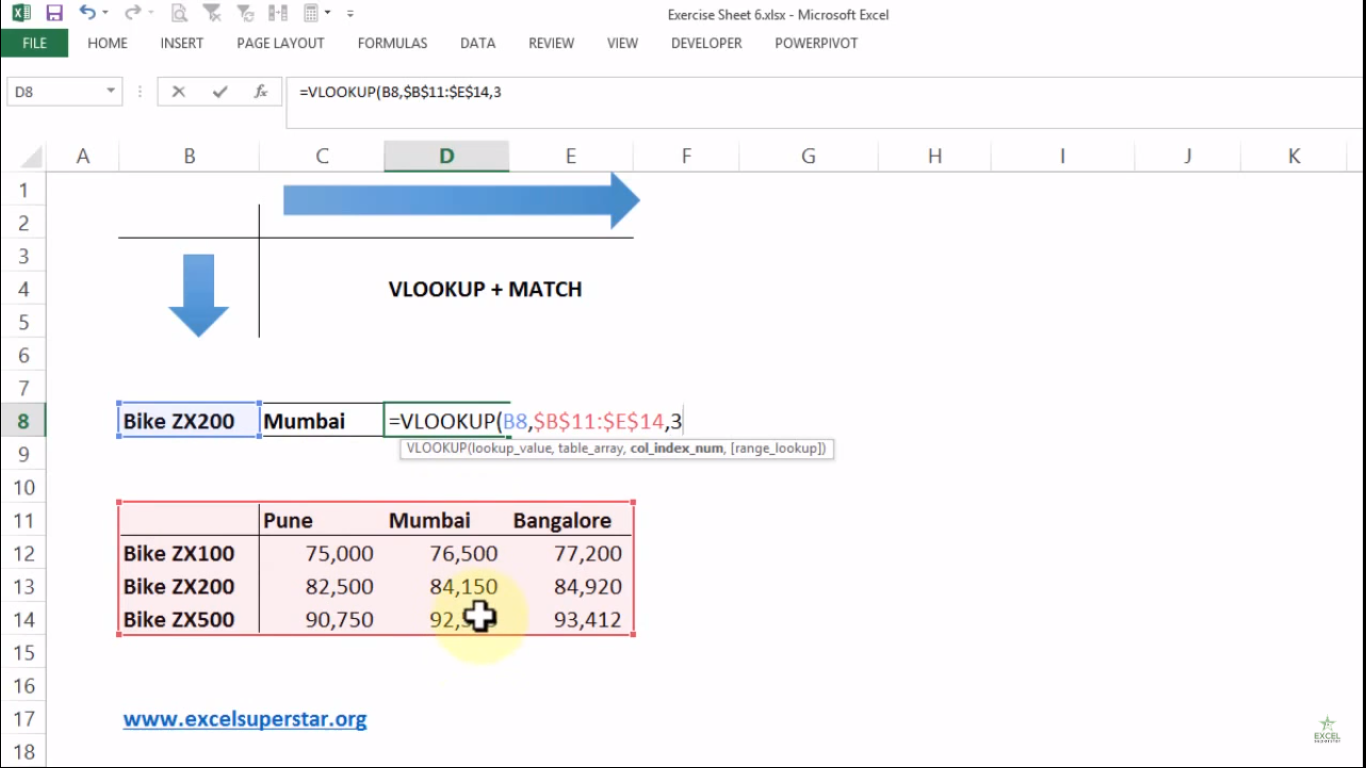Note – We want to find out the price in Mumbai city which lies in 3rd column

The fourth parameter range_lookup means TRUE = approximate match and FALSE = exact match.

5. Write FALSE or 0 to get the exact match of an answer =VLOOKUP(B8,\$B\$11:\$E\$14,3,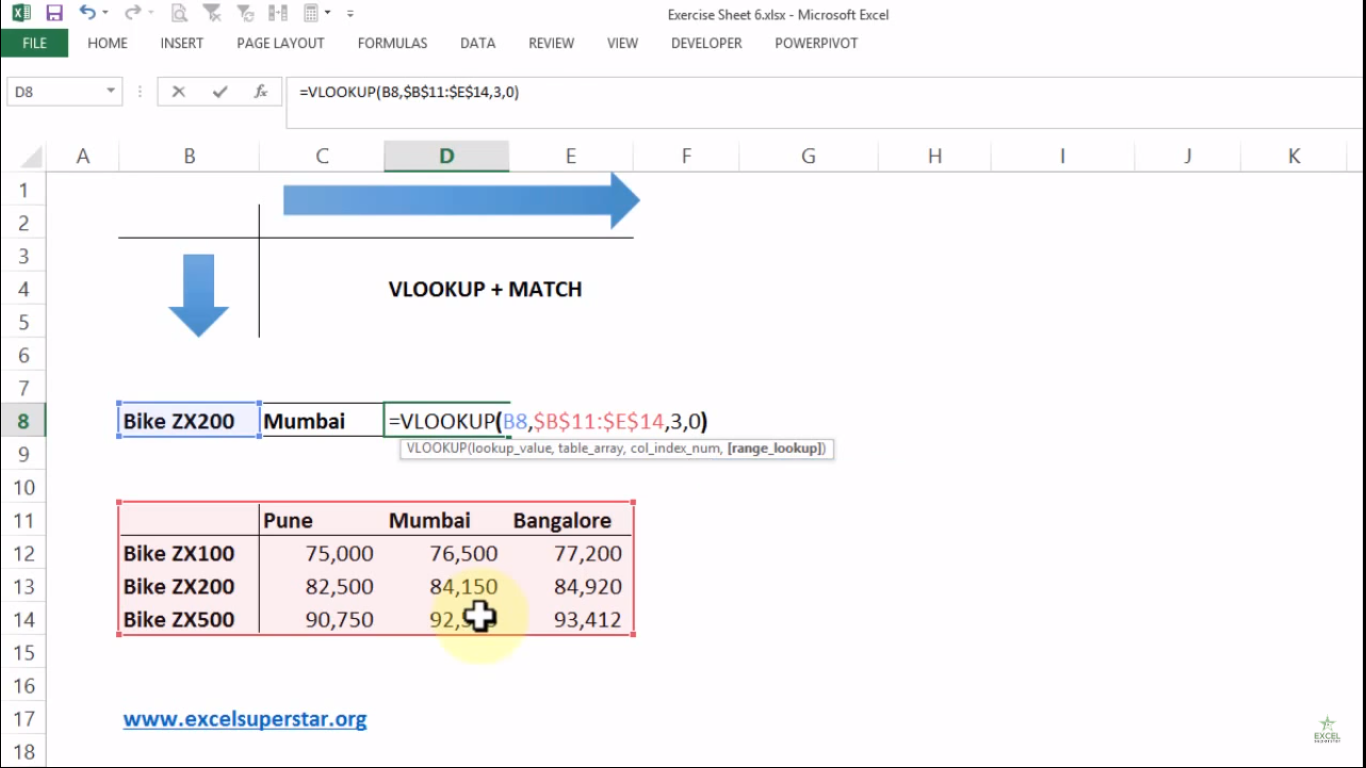6. Close the bracket and press Enter =VLOOKUP(B8,\$B\$11:\$E\$14,3,)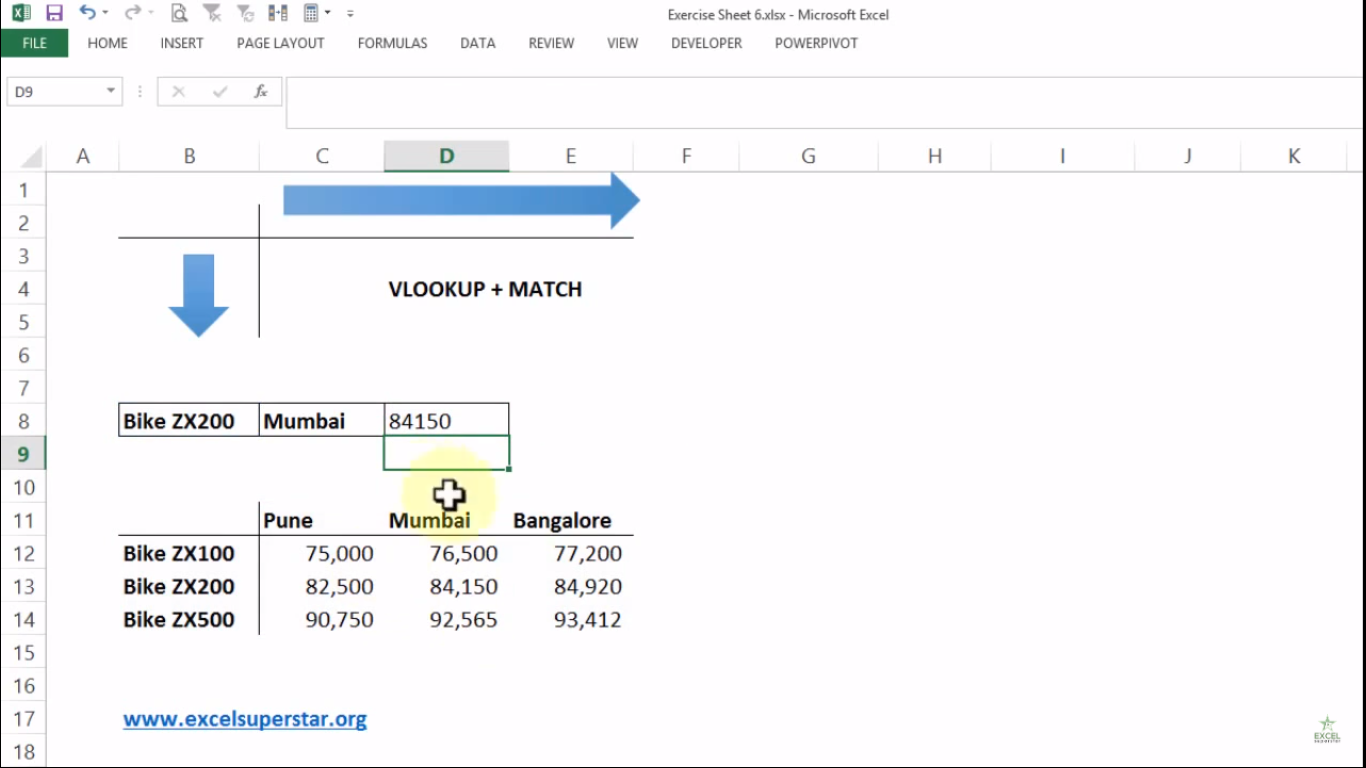Now the problem lies when you change the City from Mumbai to Pune to know the price of Bike ZX200 model then the price will not get changed because you will get the prices as per column number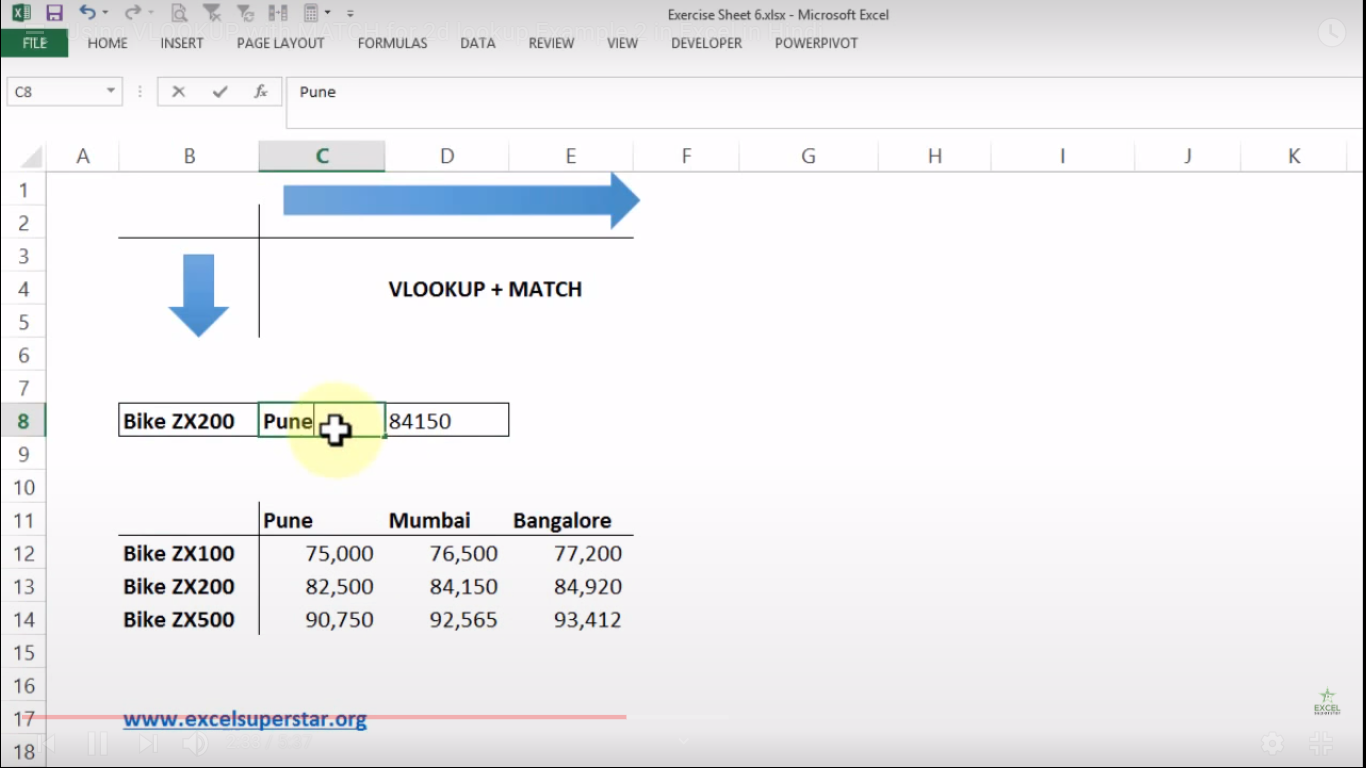So inside the VLOOKUP formula, we will remove the col_index_num which was applied before and instead, we will apply MATCH Formula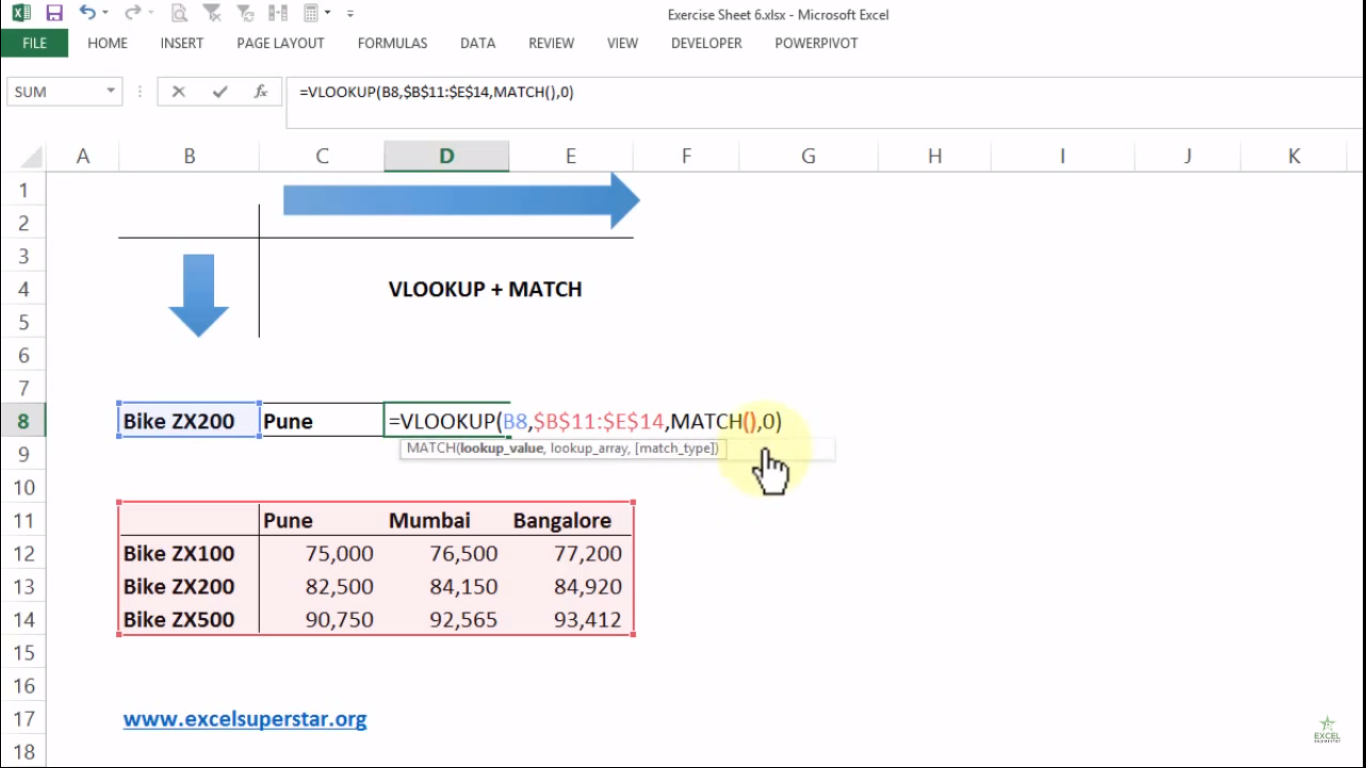Note – MATCH Formula will help us to find out the answer from Mumbai, Pune and Bangalore city which is in 2, 3 and 4 column in the given table

1. Press =MATCH

Now there are 3 parameters (lookup_value, lookup_array, [match_type])

The first parameter lookup_value means the value to match in lookup_array.

2. Select the Pune cell =MATCH(C8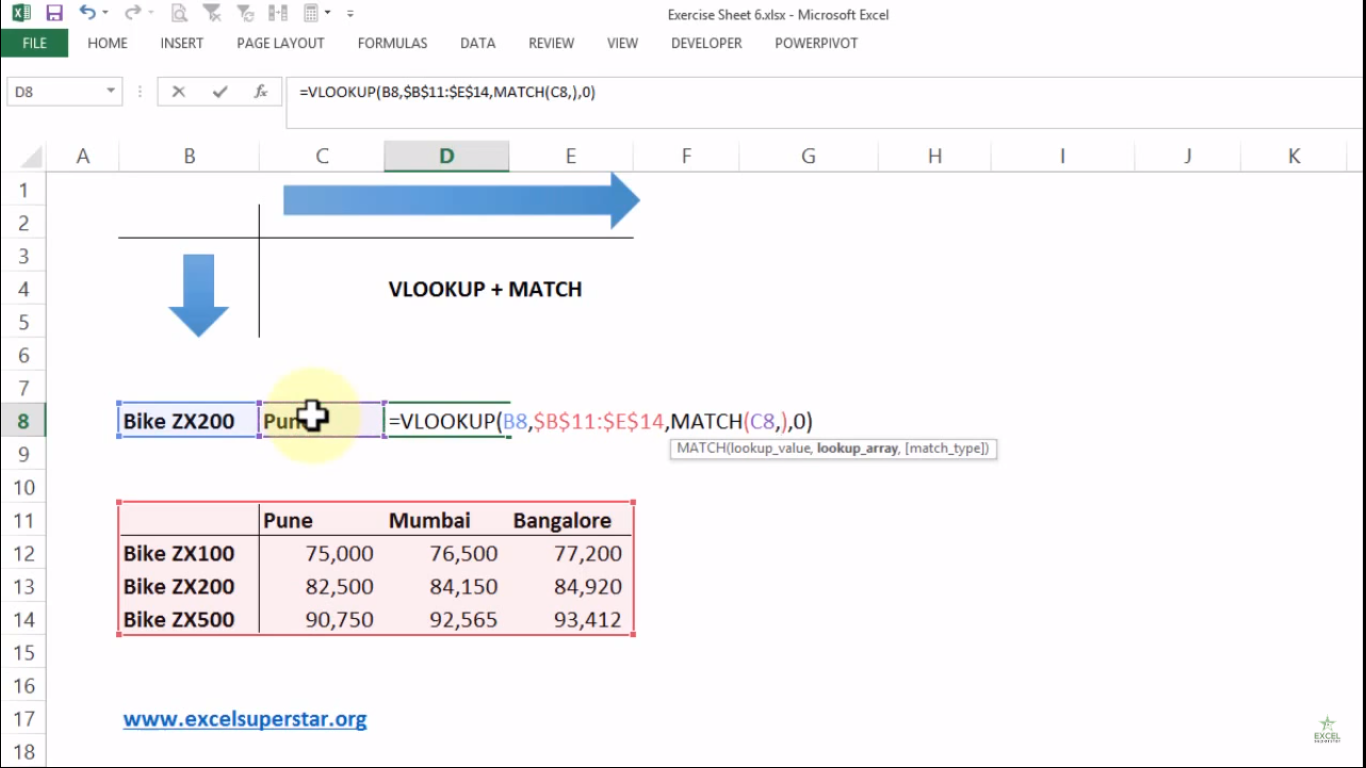The second parameter lookup_array means range of cells

3. Select the headers from the given table and press F4 =MATCH(C8, \$B\$11:\$E\$11,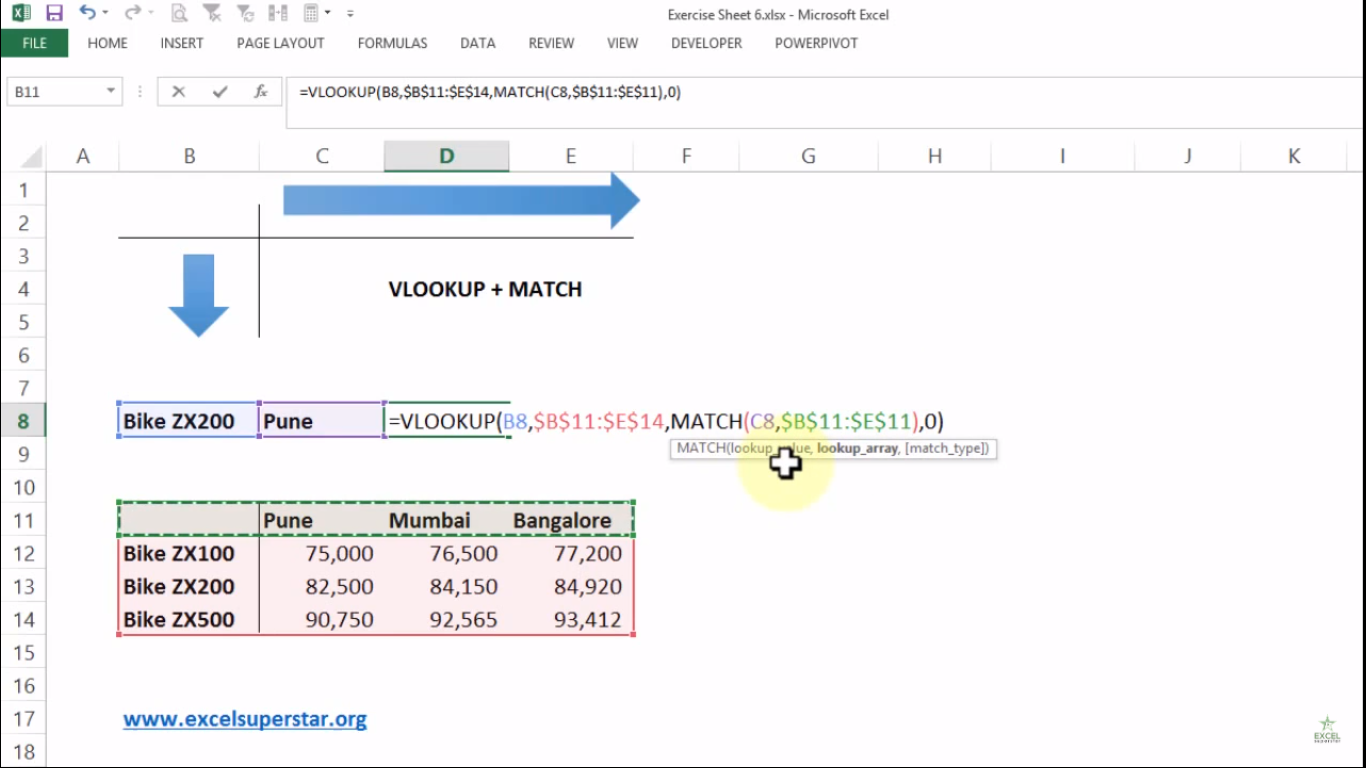The third parameter match_type means 1 =less than 0 = exact match, -1 = greater than

4. Write 0 as exact match and close the bracket =MATCH(C8, \$B\$11:\$E\$11,0)